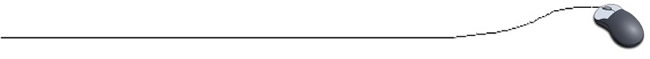Shell SortThe shell sort is named after its inventor D. L. Shell.  Instead of comparing adjacent elements, like the bubble sort, the shell sort repeatedly compares elements that are a certain distance away from each other (d represents this distance).  The value of d starts out as half the input size and is halved after each pass through the array.  The elements are compared and swapped when needed.  The equation   d = (N + 1) / 2  is used.  Notice that only integer values are used for d since integer division is occurring.
Let's look at our same list of values for descending order with the shell sort.  Remember, a "pass" is defined as one full trip through the array comparing and if necessary, swapping elements.
 Array at beginning: 84 69 76 86 94 91 d After Pass #1: 86 94 91 84 69 76 3 After Pass #2: 91 94 86 84 69 76 2 After Pass #3: 94 91 86 84 76 69 1 After Pass #4 (done): 94 91 86 84 76 69 1

First Pass:  d = (6 + 1) / 2 = 3.  Compare 1st and 4th , 2nd and 5th, and 3rd and 6th items since they are  3 positions away from each other))
Second Pass:  value for d is halved  d = (3 + 1) / 2 = 2.  Compare items two places away such as 1st and 3rd ……
Third Pass:  value for d is halved  d = (2 + 1) / 2 = 1.  Compare items one place away such as 1st and 2nd …..
Last Pass:  sort continues until d = 1 and the pass occurs without any swaps.

This sorting process, with its comparison model, is an efficient sorting algorithm.

//Shell Sort Method for Descending Order
public static void ShellSort( int [ ] num)
{
int i, temp;
boolean flag = true;

int d = num.length;
while( flag || (d > 1))
// boolean flag set to true)
{
flag = false;
// reset flag to false to check for future swaps
d = (d+1) / 2;
for (i = 0; i < (num.length - d); i++)
{
if (num[i + d] > num[i])
{
temp = num[i + d];
// swap positions i+d and i
num[i + d] = num[i];
num[i] = temp;
flag = true;
// tells swap has occurred
}
}
}
}